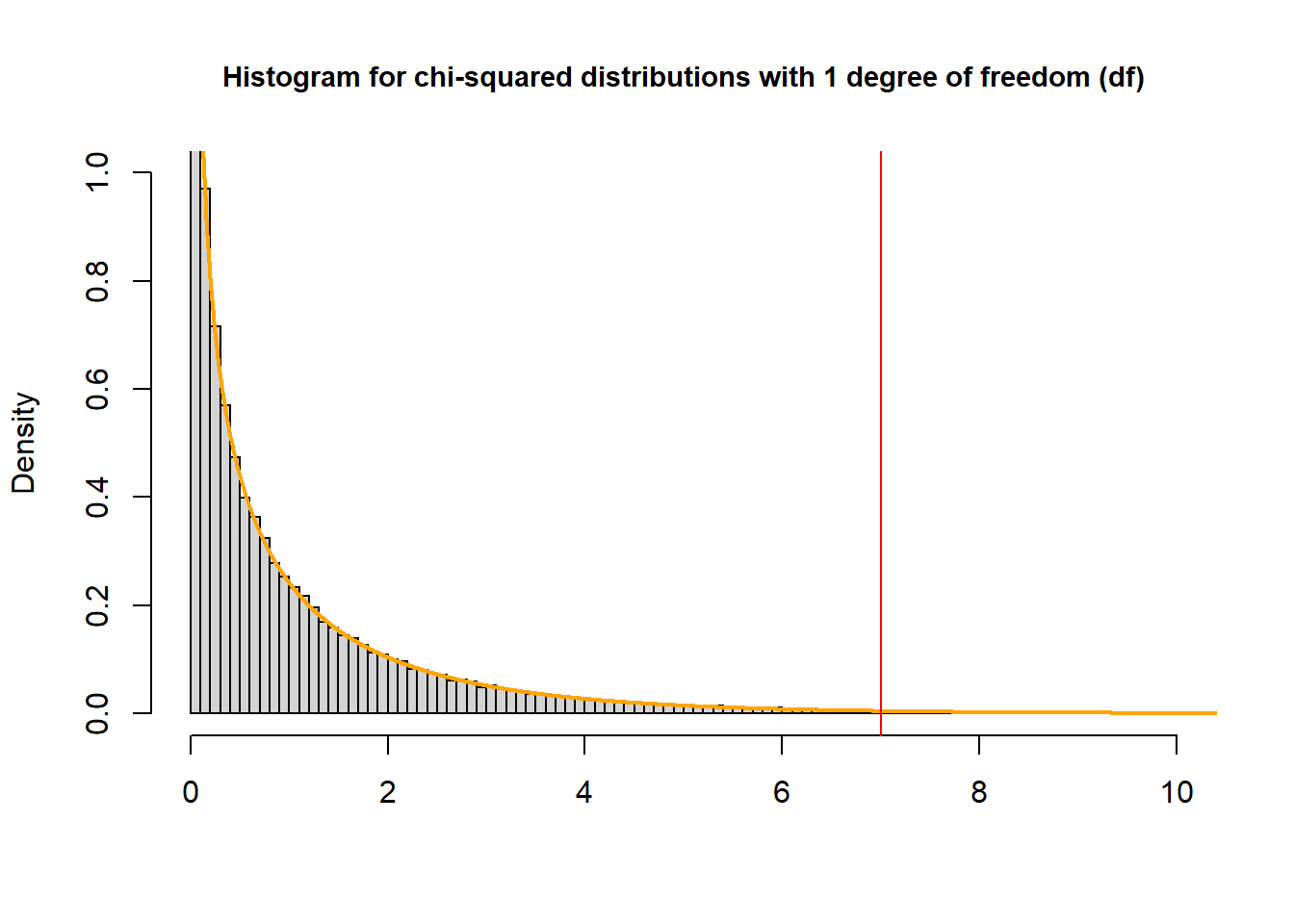# Chapter 4 Chi-squared Distribution and Tests

## 4.1chisq.test

• performs chi-squared contingency table tests and goodness-of-fit tests.
chisq.test(x, y = NULL, correct = TRUE,
p = rep(1/length(x), length(x)), rescale.p = FALSE,
simulate.p.value = FALSE, B = 2000)
• x, y = NULL: the input can be two numeric vectors as x and y (can both be factors), or a matrix as x.

• correct: a logical indicating whether to apply continuity correction.

### 4.1.1 output components

• Dataset
# Example in A01
birthwt <- data.frame("Low" = c(21054, 27126), "Normal" = c(14442, 3804294), row.names = c("Dead at Year 1", "Alive at Year 1"))
birthwt
##                   Low  Normal
## Dead at Year 1  21054   14442
## Alive at Year 1 27126 3804294
chi <- chisq.test(birthwt)
chi
##
##  Pearson's Chi-squared test with Yates' continuity correction
##
## data:  birthwt
## X-squared = 981695, df = 1, p-value < 2.2e-16
# use dollar sign($) to specify output chi$statistic # the value the chi-squared test statistic
## X-squared
##  981695.2
chi$parameter # the degrees of freedom ## df ## 1 chi$p.value
##  0
chi$method ##  "Pearson's Chi-squared test with Yates' continuity correction" chi$data.name
##  "birthwt"
chi$observed ## Low Normal ## Dead at Year 1 21054 14442 ## Alive at Year 1 27126 3804294 chi$expected  # the expected counts under the null hypothesis
##                        Low     Normal
## Dead at Year 1    442.2639   35053.74
## Alive at Year 1 47737.7361 3783682.26
chi$residuals # the Pearson residuals, (observed - expected) / sqrt(expected). ## Low Normal ## Dead at Year 1 980.10778 -110.08988 ## Alive at Year 1 -94.33735 10.59637 chi$stdres    # standardized residuals, (observed - expected) / sqrt(V)
##                       Low    Normal
## Dead at Year 1   990.8294 -990.8294
## Alive at Year 1 -990.8294  990.8294

## 4.2pchisq

• Density, distribution function, quantile function and random generation for the chi-squared ($$\chi^2$$) distribution with df degrees of freedom and optional non-centrality parameter ncp.
pchisq(q, df, ncp = 0, lower.tail = TRUE, log.p = FALSE)
• q: (vector of) quantile(s).

• df: degrees of freedom (non-negative, but can be non-integer).

• lower.tail: logical; if TRUE (default), probabilities are $$P[X \le x]$$, otherwise, $$P[X > x]$$.

### 4.2.1 Example

To find the p-value that corresponds with a $$\chi^2$$ test statistic of 7 from a test with one degree of freedom:

pchisq(7, df=1, lower.tail = FALSE)
##  0.008150972• The P-value is the probability of observing a sample statistic as extreme as the test statistic – the area to the left of the red line – “upper” tail

• We always set lower.tail = FALSE when calculating P-value of $$\chi^2$$ test.

• Another method to get the p-value

# default: lower.tail = TURE
1 - pchisq(7, df=1)
##  0.008150972

## 4.3mantelhaen.test

Performs a Cochran-Mantel-Haenszel chi-squared test of the null that two nominal variables are conditionally independent in each stratum, assuming that there is no three-way interaction.

• To test the null hypothesis that the exposure is independent of the disease when adjusting for confounding, we can use
mantelhaen.test(x, y = NULL, z = NULL,
alternative = c("two.sided", "less", "greater"),
correct = TRUE, exact = FALSE, conf.level = 0.95)
• Input data:

• A 3-dimensional contingency table in array form as x
• Or three factor objects with at least 2 levels as x, y, and z.
• correct = TRUE: Whether to apply continuity correction when computing the test statistic.

• exact = FALSE: Whether the Mantel-Haenszel test or the exact conditional test (given the strata margins) should be computed.

# example
tab <- with(dat, table(diet, mort, by = act))
mantelhaen.test(tab)
##
##         0.7851281